Shipra Saxena — Updated On April 6th, 2021

## Objective

• Bayes theorem gives the probability of an event based on the prior knowledge of conditions.
• Understand the basics of probability, conditional probability, and Bayes theorem.

## Introduction

Naive Bayes is a probabilistic algorithm. In this case, we try to calculate the probability of each class for each observation. For example, if we have two classes C1 and C2. For every observation naive Bayes calculates the probability that the observation belongs to class 1 and the probability that the observation belongs to class 2. Whichever probability is higher is selected as the class of that observation.

Note: If you are more interested in learning concepts in an Audio-Visual format, We have this entire article explained in the video below. If not, you may continue reading.

To understand the concept of Naive Bayes you must be familiar with the following concepts.

• Probability
• Conditional Probability
• Bayes Theorem

## Probability

Probability measures the likelihood that an event will occur or the chances of the occurrence of an event. This value lies between 0 and 1.

If the probability of an event is closer to 0 then we can say, the chances of occurrence of this event are very low. On the other hand, if the probability of occurrence of an event is closer to 1 then we can say, the chances of occurrence of this event are very high.

The probability of any event is calculated in the following manner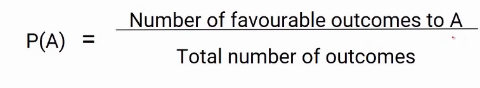We consider the number of favorable outcomes of the event and divide it by the total number of outcomes which is often called the sample space.

Let’s take a few examples, suppose we want to calculate the probability of a head when we toss a coin.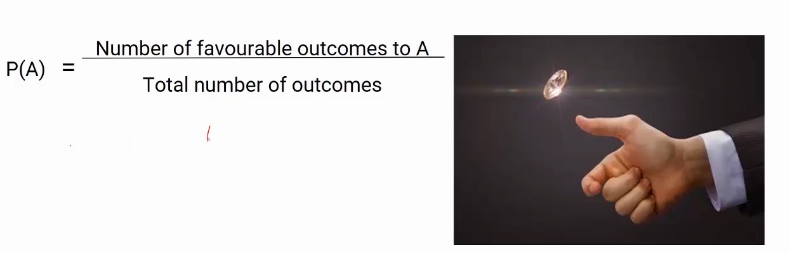In this case, we have two possible outcomes Head and Tail. Also, we have the number of the favorable outcome as one. So here, the probability is 1/2 i.e 0.5. This means the probability of getting  head when we toss an unbiased coin is 0.5

Let’s take another example. Suppose we want the probability of getting an even number on the roll of a dice. So in this case our event is to get an even number.On a roll of dice, we can get any number from 1 to 6. So our total number of outcomes is 6. In this case, a favorable outcome can be 2,4 or 6 i.e total favorable outcomes can be 3. Hence the probability of getting an even number will be 3/6 which again comes out to be 0.5.

## Conditional Probability

Let’s look at another concept, conditional probability. It calculates the probability of an event for a given condition. Consider an example where we roll two dice and we want to calculate the probability that the second dice will get a  value of 2 given that the sum of the numbers on both dices should be less than or equal to 5.

In this case, getting 2 on the second dice is an event and getting the sum of less than or equal to 5 is the condition. The conditional probability can be represented in the following manner.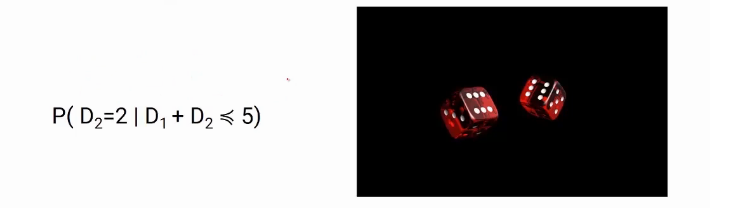Here we calculate the probability of occurrence of an event (D2=2) for a given condition (D1+ D2<=5).

If we break down this problem, we have two events. Event 1 is getting the sum of less than or equal to 5 and Event 2 is getting value 2 on the second dice. Our favorable outcome will be the intersection of the two events. The orange part in the below image represents the probability of getting event 2 when the condition of event 1 is satisfied.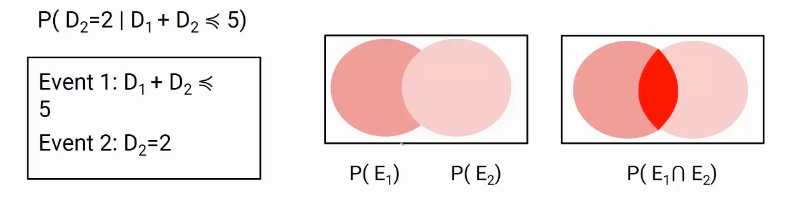So we can write the formula of conditional probability as follows.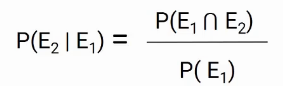The probability of E1 intersection E2 divided by the probability of E1.

Let’s use this formula in our example. Here we have all possible outcomes that we can get by throwing two dices.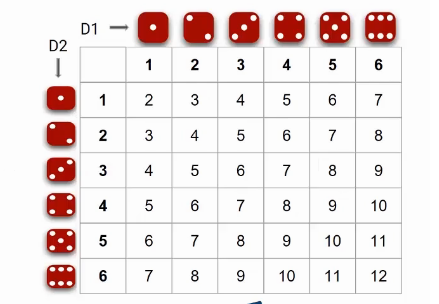Out of these 36 outcomes, what is the probability of getting a sum of less than equal to 5? We have 10 numbers as shown below which have sum less than equal to 5. So the probability of event E1 can be written as 10/36.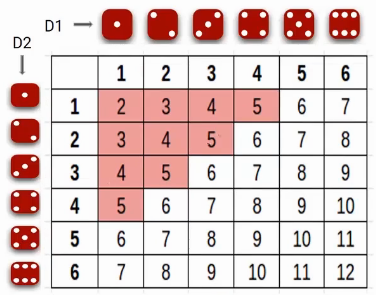Now let’s find out the probability of E1 intersection E2. So out of those 10 cases, what are the numbers where we have 2 on the second dice? We have only 3 cases that satisfy both conditions. As shown below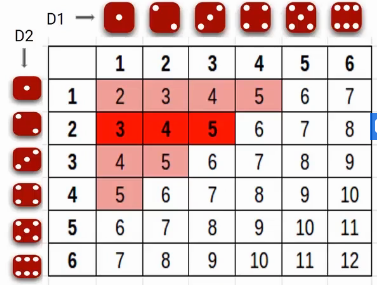So the probability of E1 intersection E2 is 3/36

## Bayes Theorem

So we have the following formula for conditional probability E1|E2Similarly, we can have the probability of E2|E1 as followsNow Probability of E1 intersection E2 will be the same in both cases so we can write it as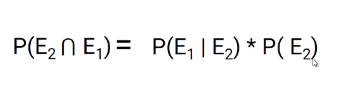Now we can replace the value in the first equation.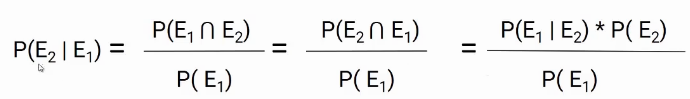The final equation comes out to be as shown in the image below.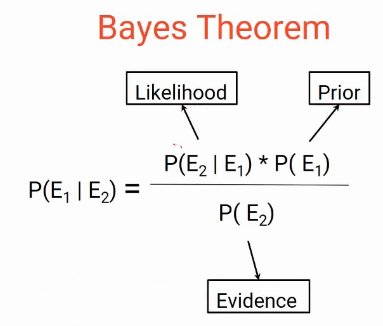This is our Bayes theorem. Where we have Likelihood, Prior, and the Evidence

## EndNotes